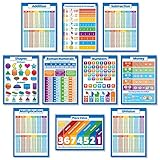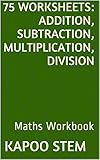Home » Posts tagged 'Subtraction'

# Tag Archives: Subtraction

## 10 Large Math Posters for Kids – Multiplication Chart, Division, Addition, Subtraction, Numbers 1-100 +, 3D Shapes, Fractions, Decimals, Percentages, Roman Numerals, Place Value, Money (PAPER) 18 x 24

### 10 Large Math Posters for Kids – Multiplication Chart, Division, Addition, Subtraction, Numbers 1-100 +, 3D Shapes, Fractions, Decimals, Percentages, Roman Numerals, Place Value, Money (PAPER) 18 x 24• 10 Educational Math Posters for Kids – Multiplication Chart, Division, Addition, Subtraction, Numbers 1-100 +, 3D Shapes, Fractions, Decimals, Percentages, Roman Numerals, Place Value
• 120 lb Poster Paper (NON – LAMINATED)

10 Educational Math Posters for Kids – Multiplication Chart, Division, Addition, Subtraction, Numbers 1-100 +, 3D Shapes, Fractions, Decimals, Percentages, Roman Numerals, Place Value

List Price: \$ 19.95

Price: \$ 19.95

## 75 Worksheets for Daily Math Practice: Addition, Subtraction, Multiplication, Division: Maths Workbook

### 75 Worksheets for Daily Math Practice: Addition, Subtraction, Multiplication, Division: Maths Workbook## Daily Math Practice 75 Worksheets

This e-book contains several math worksheets for practice. There is one worksheet for each type of math problem including different digits with operations of addition, subtraction, multiplication and division. These varying level of mathematical ability activities help in improving adding, subtracting, multiplying and dividing operation skills of the student by frequent practicing of the worksheets provided.

There is nothing more effective than a pencil and paper for practicing some math skills. These math worksheets are ideal for teachers, parents, students, and home schoolers. The companion ebook allows you to take print outs of these worksheets instantly or you can save them for later use. The learner can significantly improve math knowledge by developing a simple habit to daily practice the math drills.

Tutors and homeschoolers use the maths worksheets to test and measure the child’s mastery of basic math skills. These math drill sheets can save you precious planning time when homeschooling as you can use these work sheets to give extra practice of essential math skills. Parents use these mathematics worksheets for their kids homework practice too.

Designed for after school study and self study, it is used by homeschooler, special needs and gifted kids to add to the learning experience in positive ways. You can also use the worksheets during the summer to get your children ready for the upcoming school term. It helps your child excel in school as well as in building good study habits. If a workbook or mathematic textbook is not allowing for much basic practise, these sheets give you the flexibility to follow the practice that your student needs for an education curriculum.

These worksheets are not designed to be grade specific for students, rather depend on how much practice they’ve had at the skill in the past and how the curriculum in your school is organized. Kids work at their own level and their own pace through these activities. The learner can practice one worksheet a day, two worksheets a day, one every alternate day, one per week, two per week or can follow any consistent pattern. Make best use of your judgement.

Price: